### Gaurang Paranji

Gaurang Paranji Python Portfolio

Gaurang Paranji

# Report 1: Primitive Pythagorean Triple¶

Gaurang Paranji

## Primitive Pythagorean Triples¶

Primitive Pythagorean Triples are positive integers such that $a^2 + b^2 = c^2$, where a, b, and c are relatively prime.

The required task was to generate all Primitive Pythagorean Triples for $a,b<=0$. To do this, I had to do three things.

1. I had to check whether the greatest common divisor for a and b was 1. I did this by expressing the Euclidean Algorithm in Python.
2. Then I had to check whether the sum of $a^2$ and $b^2$ was a perfect square. I did this by taking the square root of the sum, converting it to an int and then multiplying it by itself to see if the product was exactly c. If it wasn’t, then I could safely say that the root wasn’t an integer, and therefore c wasn’t a perfect square.
3. Then, to confirm that two numbers were indeed Primitive Pythagorean Triples I had to write a function that checked if:

a) the greatest common divisor was 1; and

b) the sum of the squares of the two numbers was prime

If I was sure of those two conditions, I could then go ahead and append the numbers to a list of the triples. I also added a and b to individual lists so I could go on to plot them.

## Analysis¶

The Primitive Pythagorean Triples seem to be radiating outwards in a floral pattern.

In :
%pylab inline
def mygcd(f,g):
while f!=g:
if f>g:
f-=g
else:
g-=f
return(f)

def is_square(x):
y= int(x**(0.5))
return (y*y)==x

def PPTFinder(a,b):
t=((a**2)+(b**2))
return mygcd(a,b)==1 and is_square(t)

def PPTPlotter(pt):
triplets = []
iare = []
jare = []
for i in range(1,pt+1):
for j in range(1,pt+1):
if PPTFinder(i,j):
k = (i**2)+(j**2)
triplets.append([i,j,k])
iare.append(i)
jare.append(j)
##print(triplets)
figure(figsize=(12,8))
plot(iare,jare,'r.',ms=10)
title("Primitive Pythagorean Triples")

Populating the interactive namespace from numpy and matplotlib

In :
PPTPlotter(5000) ##range you want to go upto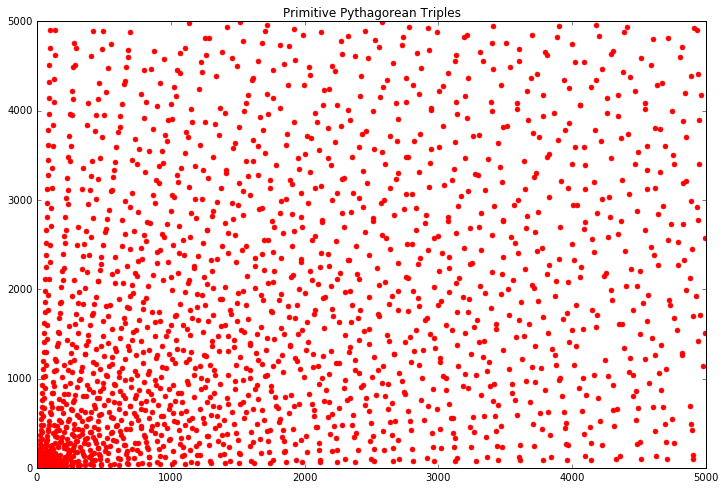In [ ]:



# Report 2: Prime Spirals

Gaurang Paranji

For this assignment we had to code in Python a version of the spiral that mathematician Stanislaw Ulam first drew in 1963. The spiral is centered on the number 1, and the numbers radiate outwards as they increase. To make things interesting, he decided to highlight prime numbers as he came across them. Ulam then decided to produce this spiral up to 65,000 with the help of a computer. At this point patterns began to appear, which is what makes Ulam’s Spiral Notable.

## The Process

To code the Spiral, I used three nested loops: initial variables:

1. *sidelength*: This is the length of each side less one, so I can control how many numbers are printed.
2. *nextmove*: This is a list containing all possible moves in the Spiral.
3. *nextmoveindex*: Initialising the starting index of *nextmove*.
4. *wtp*: For "where to print;" Stores the direction in which to print the next number.
5. *nosprinted*: To control the numbers printed on a side.
6. *xindex,yindex*: Initial x and y axis indexes.


### *is_prime*

This function checks to see whether a given number is prime or not by returning False the moment it finds a perfect divisor, True otherwise.

### *moveincrementer*

To increment the x and y indexes in the required direction.

### Loop 1:

This loop was to ensure the incrementor hadn’t gotten too big, while also making sure that numbers were generated at least to a certain point.

### Loop 2:

One of the properties of the spiral is that there are two sides of equal length. This loop finished one cycle, changed the direction in which the numbers printed, and ran again to complete the second side of the same length. The variable that dictated the length of the side was then incremented by 1.

### Loop 3:

This loop was the one that actually handled the printing of the numbers. It would first increment the x and y indexes as required by calling the function moveincrementor in the direction which was stored in the variable wtp The variable wtp is then incremented after the completion of one side. The next direction to go in is stored in a list nextmove. Once the index of nextmove reaches 3 (the final occupied index), I wrote an if statement to loop it back to 0. The code in this loop then checks if the number is prime by calling the function is_prime. If it was prime, it printed the number in red, otherwise blue. Once the number has printed, it is incremented and there is a check to see if we have reached the requisite number yet. If not, the loop was allowed to continue.

## The Code:

In :
%pylab inline

sidelength=1
nextmove = ['r','u','l','d']
nextmoveindex = 0
wtp = 'r'
nosprinted = 0
xindex,yindex=0,0

def is_prime(p):
for i in range(2,p):
if p%i==0:
return False
return True

def moveincrementor(xindex,yindex):
if wtp == 'r':
xindex=xindex+4
if wtp == 'l':
xindex=xindex-4
if wtp == 'u':
yindex=yindex+4
if wtp == 'd':
yindex=yindex-4

return xindex,yindex

def wtpindexincrementor(nextmoveindex):
if nextmoveindex!=3:
nextmoveindex=nextmoveindex+1

else:
nextmoveindex=0

return nextmoveindex

text(xindex,yindex,1,color='r')

i=2
while i<900:
for j in range(1,3):
while nosprinted!=sidelength:
xindex,yindex=moveincrementor(xindex,yindex)
if is_prime(i):
text(xindex, yindex, i, color='r')
else:
text(xindex, yindex, i, color='b')
if i<900:
i=i+1
else:
break
nosprinted=nosprinted+1

xlim(-30,30)
ylim(-30,30)

nosprinted = 0
nextmoveindex=wtpindexincrementor(nextmoveindex)
wtp = nextmove[nextmoveindex]

sidelength=sidelength+1
plt.axis('off')

Populating the interactive namespace from numpy and matplotlib

Out:
(-30.0, 30.0, -30.0, 30.0)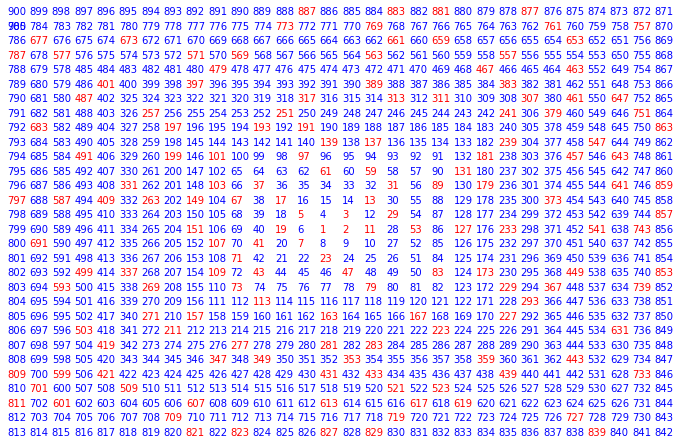## Analysis

Perhaps the most obvious is that there is a pattern of diagonals running both left to right and right to left. Horizontal and Vertical lines are also evident. This is possible because most prime numbers are odd. This means that every other diagonal will most certainly not be prime as they contain even numbers, which are deivisible by 2.

# Report 4: Floating Point Numbers

Gaurang Paranji

The task for this report was to experiment with Python to see how floating point numbers are represented in it. We look at Machine Epsilon, and the largest and smallest floating point numbers that can be stored.

In :
%pylab inline

Populating the interactive namespace from numpy and matplotlib


## Exercise 1¶

Here we write a function to find Machine Epsilon, $\epsilon$ , which is the relative increase from one floating point to the other. It is the maximum relative error you can get due to rounding in floating point arithmetic. To find $\epsilon$, we increment x and its exponent n until the sum of x and 1 is not equal to 1. At this point the addition function breaks, and the previous n value is the last possible power of 2.

In :
def find_epsilon():
n=1
x= 2.**(-n)
while 1+x != 1:
n+=1
x=2.**-n
return n-1


## Exercise 2¶

This function was defined to find the largest possible floating point number that can be stored, and it prints the exponent to reach this number. When the number is exceeded, python throws an exception and the loop is exited after the except block prints out the previous exponent

In :
def find_largest():
n=1
while True:
try:
x=2.**n
n+=1
except:
return(n-1)
break


## Exercise 3¶

The function to find the smallest value is similar to the one to find machine epsilon. It is different from the find largest function because once the exponent for the smallest float is reached, meaning the smallest representable number is found, because Python automatically truncates the number to 0, so no error would be thrown.

In :
def find_smallest():
n=1
x= 2.**(n)
while x != 0:
n-=1
x=2.**(n)
return(n-1)


## Exercise 4¶

This exercise is to show how truncation errors occurs due to the addition in $log(1+x)/x$. Close to $\epsilon$, $1+x$ starts to get truncated due to how floating points are represented and we end up with the anomalies seen on the graph.

In :
figsize(10,6)
end = 1e-15
x = linspace(-end,end,1001)
y = where(x==0,1.0,log(1+x)/x)
plot(x,y);

C:\Anaconda3\lib\site-packages\ipykernel\__main__.py:4: RuntimeWarning: invalid value encountered in true_divide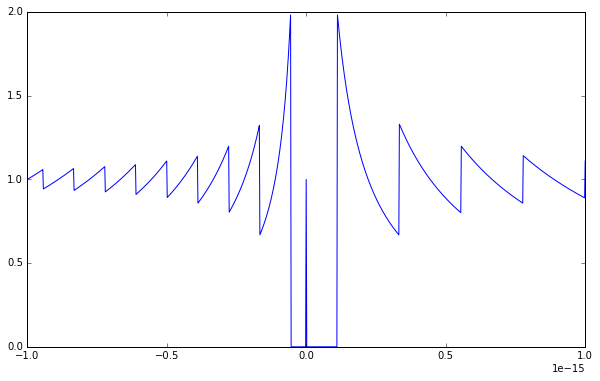## Exercise 5¶

In this exercise, we use Taylor Series Approximations instead of using the log function directly. This has the benefit of using division instead of addition, which does not result in as many floating point errors.

The Taylor series expansion for the function $g(x)$ is given by $$g(x) = 1 – \frac{x}{2} + \frac{x^2}{3} – \frac{x^3}{4} + \frac{x^2}{5}$$

In :
figsize(10,6)
end = 1e-15
x = linspace(-end,end,1001)
y = where(x==0,1.0,log(1+x)/x)
plot(x,y);

y2 = where(x==0,1.0,1-(x/2)+((x**2)/3)-((x**3)/4)+((x**5)/5))
plot(x,y2,label="Taylor Series Approx.")

legend();

C:\Anaconda3\lib\site-packages\ipykernel\__main__.py:4: RuntimeWarning: invalid value encountered in true_divide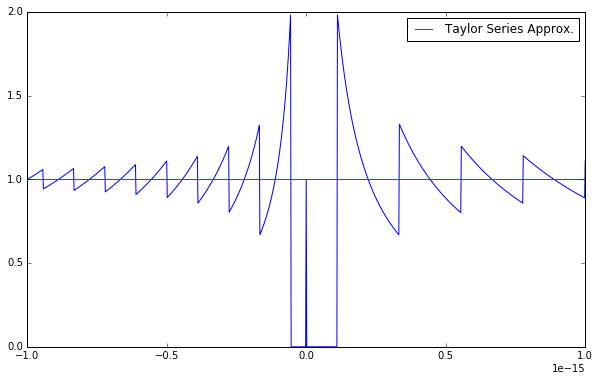## Analysis

The value of $\epsilon$ is:

In :
print(2**-(find_epsilon()))

2.220446049250313e-16


The bias is:

In :
-(find_smallest())-(find_largest())

Out:
54

# Report 5: Cellular Automata

Gaurang Paranji

For this assignment we had to design a “self-contained stylized universe” consisting of a grid of cells that had values based on the values of their neighbors, meaning that they are local

In :
%pylab inline
from numpy.random import randint

Populating the interactive namespace from numpy and matplotlib


The cells have four possible values: 0,1,2, or 3. The values are randomized with the function random.randint imported from NumPy. The transitions rules are the uniform “laws” that all cells must follow.

This function returns a randomly generated transition rule every time it is invoked.

In :
def transition_rule():
trule_size = 100
trule = arange(trule_size) #creates an array of size trule_size

#fills trule with random integers.
for i in range(trule_size):
trule[i] = random.randint(0,4)
return(trule)


We randomizing the first row of cell states which are stored in a 2D integer array. We do this so each subsequent row of cells can have their values, “states” based upon their preceding row depending on the transition rules.

This function returns the cell states which can then be plotted into an image.

In :
def cell_states(trule):
noc = 100
nogens = 100
cstates = empty((nogens,noc),dtype=int)

#fills the first row with random integers
for columns in range(0,noc):
cstates[0,columns] = random.randint(0,4)

#calculates the cell state for each subsequent row
for rows in range(1,nogens):
prev = cstates[rows-1]
total=prev+roll(prev,1)+roll(prev,-1)
cstates[rows] = trule[total]

return(cstates)


This is the list of colors that I use to plot each cell state into an image. The three values of each row of floats are the RGB values for a certain color, represented as floats in the interval [0,1]. Each row corresponds to a different cell state.

In :
colors=empty((4,3),dtype=float)
colors=95,65,217
colors= 217,156,65
colors= 187,217,65
colors= 217,65,187
colors /= 255.


This is where I generate and plot the images. Each image is in a subplot with it’s axes turned off, and titled with their index number, which I converted from an int to a string.

In :
rows=5
cols=5
for k in range(1,rows*cols+1):
plot_array = cell_states(transition_rule())
figsize(25,25)
subplot(rows,cols,k)
title(str(k))
axis("off")
imshow(colors[plot_array], interpolation = 'none');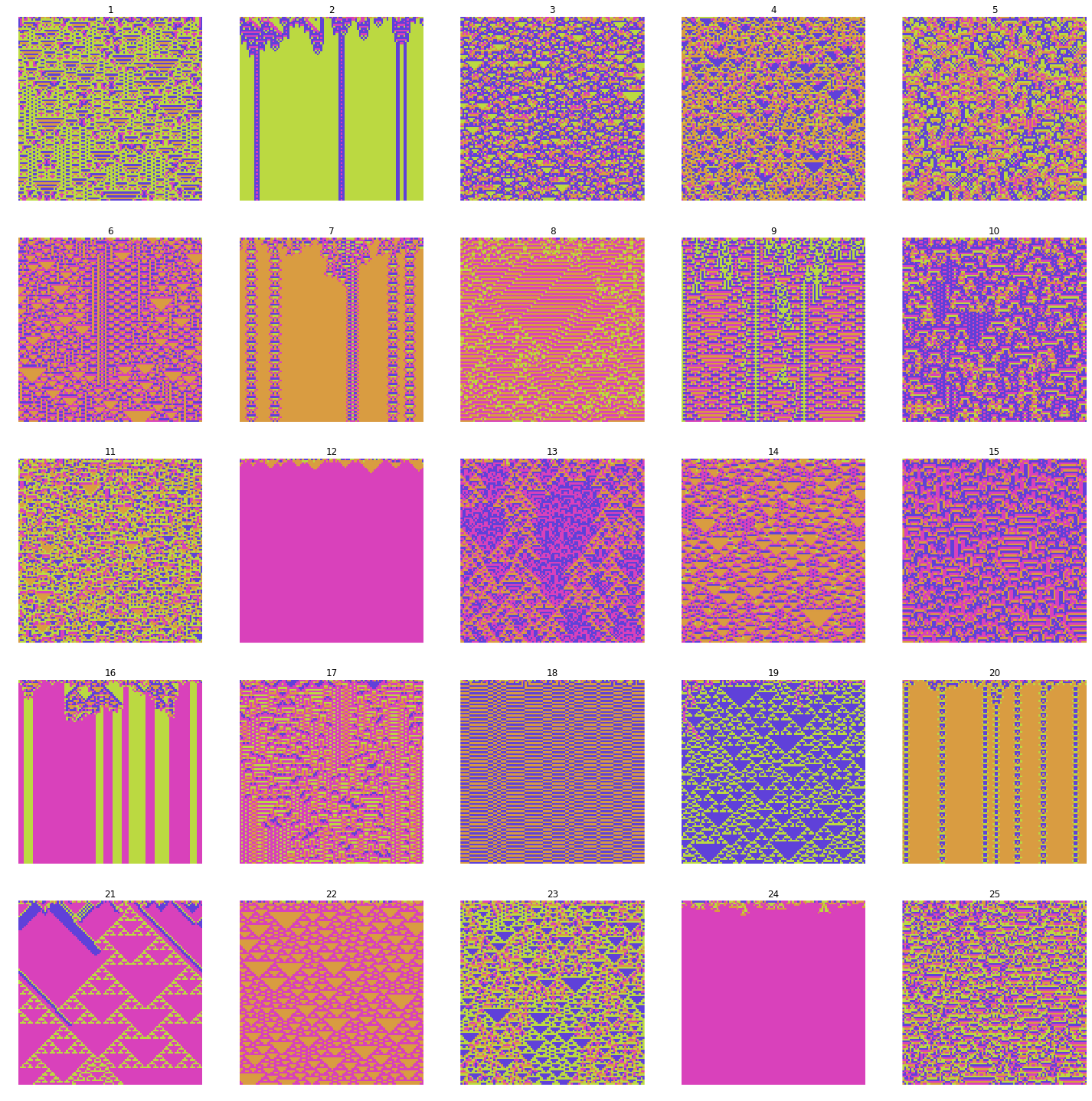## Analysis

Their seem to be five main automata types:

1. **Inverted Pyramid:** Most common, including images 1-5, 9-11, 20 and 23.
2. **Trees:** Shaped like binary trees, the only example of this being 24. I saw a lot more like these on previous iterations.
3. **Solid Colors:** Including 6, 8, 16, 17 and 21.
4: **Striations:** 17, 18 and 25 show these vertical striations most prominently.
5: **Mixed:** These mix the above our types. Examples are 15 and 24, showing a mix of the *tree* and *striation* types; and number 19, showing *inverted pyramids* and *striations*.
In [ ]:


In :
rows=5
cols=1
for k in range(1,rows*cols+1):
tr=transition_rule()
plot_array = cell_states(tr)
figsize(25,25)
subplot(rows,cols,k)
title(tr)
axis("off")
imshow(colors[plot_array], interpolation = 'none');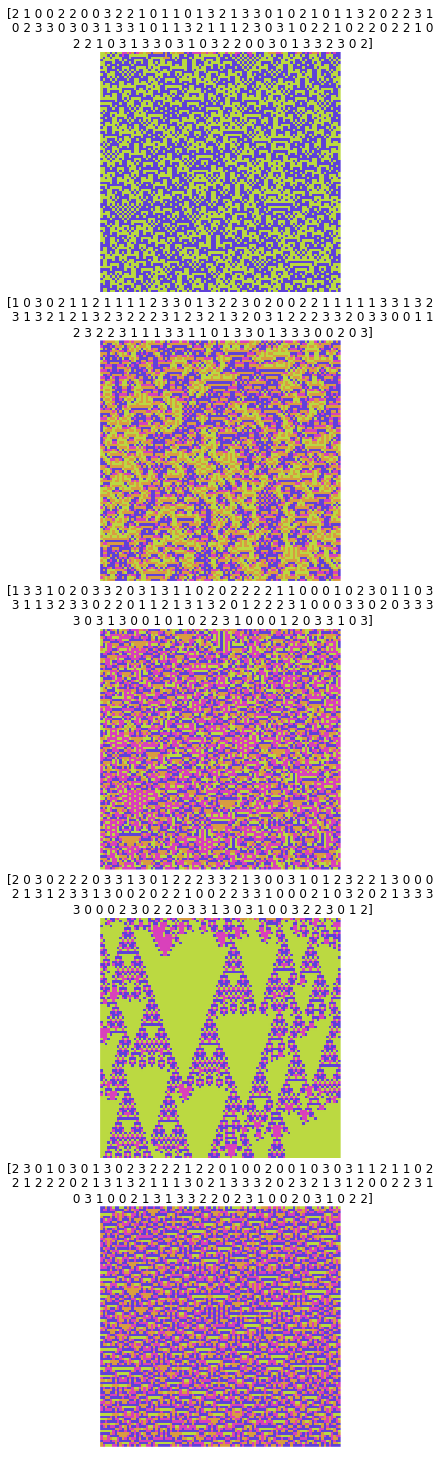# Report 6: The Magnetic Pendulum

Gaurang Paranji

The task involves simulating the motion of a pendulum that is suspended from a pivot at the origin, the motion of which is affected by magnets at a given position. The state of the system is given my a matrix $$\overrightarrow{P} = \begin{bmatrix} x \\ y \\ v_x \\v_y \end{bmatrix}$$ where $(x,y)$ is the position of the pendulum, and $v_x$ and $v_y$ are the velocities in the $\overrightarrow{x}$ and $\overrightarrow{y}$ directions respectively.

The motion of the pendulum is governed by three forces:

1. Gravity
2. Friction
3. Magnetism
In :
%pylab
from matplotlib import animation

Using matplotlib backend: Qt4Agg
Populating the interactive namespace from numpy and matplotlib


This function’s sole purpose is to return the locations of the magnetic dipoles.

In :
def magnet_locations():
return array([[1., 0.], [-.5, sqrt(3)/2], [-.5, -sqrt(3)/2]])


This function accounts for all three forces.

Friction and Gravity are simplified into a single coefficient.

Magnetism is governed by the standard formula $$r_i = |r_i| = \sqrt{(x-x_i)^2 + (y-y_i)^2 + d^2}$$ where $d$ is the distance between the magnets.

$v_x$ and $v_y$ is then given by a combination of these three forces.

In :
def dP_dt(P, C, R, d):
x, y, vx, vy = P
MAGNETS = magnet_locations()
Xs, Ys = 0, 0
for xi,yi in MAGNETS:
ri=sqrt((x-xi)**2+(y-yi)**2+d**2)
Xs+=((x-xi)/(ri**5))*(1-5*(d**2)/(ri**2))
Ys+=((y-yi)/(ri**5))*(1-5*(d**2)/(ri**2))
ax = -C*x - R*vx + Xs
ay = -C*y - R*vy + Ys
return array([vx,vy,ax,ay])

In :
def plot_path(tmax, h, P, C, R, d):
path = calculate_path(tmax, h, P , C, R, d)
plot(path[:,0], path[:,1])

In :
def calculate_path(tmax, h, P, C, R, d):
steps = int(tmax/h)
path = empty((steps,4))
path=P
for i in range(1,steps):
f1 = h*dP_dt(P, C, R, d)
f2 = h*dP_dt(P + f1, C, R, d)
P += (f1+f2) / 2.
path[i] = P
return path

In :
def calculate_final_position(tmax, h, P, C, R, d):
steps = int(tmax/h)
for i in range(1, steps):
f1 = h*dP_dt(P, C, R, d)
f2 = h*dP_dt(P + f1, C, R, d)
P+= (f1+f2)/2.
return P


## Exercise 1 & 2

We simulate the dynamics of the system using Euler’s Method. We then change the values for $C$ the constant for gravity and $R$ the constant for friction.

The pendulum is often slingshotted in a particular direction, aided by one or more magnets, especially when it passes close to said magnet(s) in a linear manner. This is similar to how spacecraft get a speed-boost or reduction by using a planet’s gravitational field. On the other hand, if the pendulum is in between the three magnets, it fails to generate any momentum and falls into the basin at the point in the center of the magnets.

In :
index = 1
d = 0.3
C = [1.,0.1,1.5,10]
R = [.1,0.01,0.5,0.1]

gca().set_aspect("equal")
mag = magnet_locations()
for P0 in (array([1.,0.,0.,1.]),array([1.,0.,0.,1.]),array([1., 0., 1., 1.]), array([0., 0., 1., 1.])):
subplot(2, 2, index)
plot(mag[:, 0], mag[:, 1], 'ko', ms=4)
plot_path(10,.1,P0,C[index-1],R[index-1],d)
index +=1



## Exercise 3

Here we use Matplotlib to animate the path taken by the pendulum.

In [ ]:
tmax = 100
h=0.1
P0 = array([1.,1.,0.,1.,])
C=1.
R=0.1
d = 0.5
path = calculate_path(tmax, h, P0, C, R, d)

frames = int(tmax/h)
fig, ax = subplots(figsize=(8,8))
ax.set_aspect('equal')
point, = plot([], [], 'bo')
line, = plot([],[],'b')

mag = magnet_locations()

i=0
while i <= len(mag)-1:
x = mag[i,0]
y = mag[i,1]
i+=1
magnetplot = plot(x,y,'ko',ms=10)

width = 0.25 + amax(abs(path[:,:2]))
xlim(-width,width)
ylim(-width, width)

def animate(frames, point, line, path):
x, y = path[frames,:2]
point.set_data(x, y)
line.set_data(path[:frames, 0], path[:frames, 1])

return point, line

animation.FuncAnimation(fig, animate, frames=frames, fargs=(point, line, path), interval =0, repeat = True)



## Exercises 4 & 5

Here we use a color coded image to show which magnet the pendulum rests on depending on the starting point. The first magnet is given by green, the second by green, and the third by blue. We then repeat this using different values of the friction constant $R$.

This image displays an almost fractal like design. There is